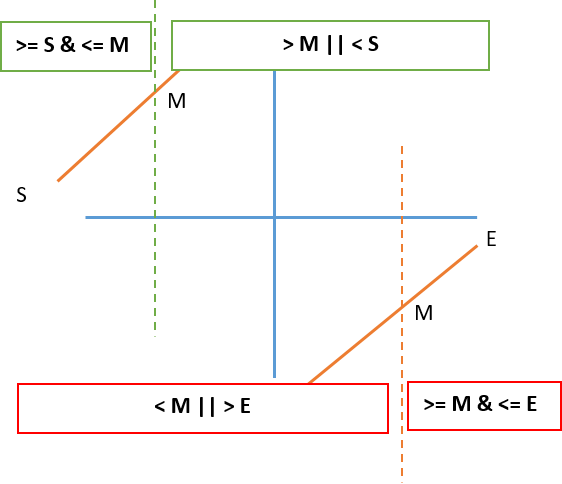So given a rotated sorted array and a target value, return its index. If target value is not found, return `-1`.

For `[4, 5, 1, 2, 3]` and target = `1`, return `2`.
For `[4, 5, 1, 2, 3]` and target = `0`, return `-1`.

Well, the question has explicitly said you need to perform a search in rotated sorted array, and by sorted we can guarantee that linear search won't work in this scenario, perhaps binary search will be more suitable. When we perform binary search, we need to take care of these two scenarios:

We need to identify these 4 case scenarios:

1. `nums[mid] > nums[start]` and `nums[start] <= target <= nums[mid]` (green case)
2. `nums[mid] > nums[start]` and `target > nums[mid] || target < nums[start]` (green case)
3. `nums[mid] < nums[end]` and `nums[mid] <= target <= nums[end]` (red case)
4. `nums[mid] < nums[end]` and `target < nums[mid] || target > nums[end]` (red case)Once we identify all the case scenarios, we can put it into code:

``````public int search(int[] nums, int target) {

if(nums == null || nums.length == 0) return -1;
int start = 0, end = nums.length - 1;

while(start + 1 < end){
int mid = start + (end - start) / 2;

if(nums[mid] == target){
return mid; // return the index
}

if(nums[start] < nums[mid]){
// green case condition
if(target >= nums[start] && target <= nums[mid]){
end = mid;
} else{
start = mid;
}
} else{
// red case condition
if(target >= nums[mid] && target <= nums[end]){
start = mid;
} else{
end = mid;
}
}
}
if(nums[start] == target) return start;
if(nums[end] == target)  return end;
return -1;
}

``````

That's it! It is not that difficult after all, right? Well, I hope this post will be a good help to you. See ya!

Post was published on , last updated on .

Like the content? Support the author by paypal.me!